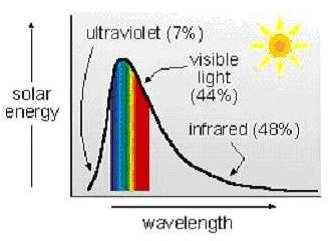# The Beer Lambert Law, also known as Lambert's Law, applied to Solar Energy Absorption by Water

Page content

## Introduction

The Lambert’s law to be discussed in this article is also referred to as the Beer Lambert law, the Lambert Beer Law, Beer’s Law, and the Beer-Lambert-Bouguer law. This law with many names is an expression of the relationship among the following parameters: i) the fraction of incident light transmitted through a substance (its transmissivity); ii) a property of the substance known as its absorption coefficient; and iii) the path length that the light travels through the material.

## Beer Lambert Law Equations

One form of the Beer Lambert Law equation, as it is typically used for liquids, is: I/Io = 10-α**l**, where:

Io = intensity of light striking a substance through which light can be transmitted, in units like W/m2 or Btu/hr/ft2,

I = intensity of light transmitted through the substance, in the same units as Io,

l = the distance that light travels through the substance, in m

α = the absorption coefficient of the substance, in m-1

Note that the fraction of the incoming light that is transmitted through the substance, I/Io, is called the transmissivity, T. Also, if none of the incident light is reflected then 1 - T is the fraction of the incoming light that is absorbed by the substance. These parameters and the Beer Lambert law equation relating them to each other are shown in the diagram above.

Another parameter, the absorbance of a liquid, A, is defined as the negative logarithm to the base 10 of the transmissivity. This leads to the following as another way of expressing Beer’s Law: A = - log10(I/Io) = α**l**. It is important to note that absorbance is not the same as absorptivity (the fraction of incident light that is absorbed by something).

## Application of Beer Lambert’s Law to Solar Energy Absorption by WaterA primary use of the Beer Lambert Law is in analytical chemistry (uv-visible spectroscopy), in which light of a selected wavelength is directed through a liquid sample in a cuvette of known pathlength. The concentration of some substance in the sample can then be determined from the measured absorbance.

Based on the variables in Beer’s Law, another application should be calculations concerning the amount of solar energy absorbed by water, as for example, an upper ocean layer of given depth, possibly in connection with ocean thermal energy conversion2 (OTEC) calculations. There are a couple of complicating factors for this application, however. Unlike the monochromatic light beam used for spectroscopy, solar radiation is made up of electromagnetic radiation over a spectrum of uv, visible and infrared wavelengths, as shown in the diagram at the right, and the absorption coefficient of the water (as with any substance) varies with the wavelength of the incident light. Stewart1 presents data showing that the absorption coefficient of pure water ranges from 1 to 10,000 cm-1 for infrared radiation and from 0.001 to 1 for uv and visible light.

Another complicating factor for applying Lambert’s Law of solar absorption of water is that the Beer Lambert Law gives the transmissivity for pure water. Ocean water contains other substances that will absorb some solar energy, and some of the incident solar energy will be reflected at the water surface and by particles in the ocean water.

## Example Beer Lambert Law Calculations

Problem Statement #1: Estimate the transmissivity of solar radiation of several wavelengths through the upper one foot layer of ocean water, using the following values for the absorption coefficient of water (from Stewart’s data1):

violet light (shortest wavelength visible light): α = 0.01 cm-1 = 0.305 ft-1

red light (longest wavelength visible light): α = 1 cm-1 = 30.5 ft-1

short wavelength infrared radiation: α = 1 cm-1 = 30.5 ft-1

long wavelength infrared radiation: α = 10,000 cm-1 = 305,000 ft-1

Solution: For violet light (α = 0.01 cm-1): T = I/Io = 10-α**l** = 10-(0.305*1) = 0.495

For red light and the shortest wavelength infrared radiation (α = 1 cm-1): T = I/Io = 10-α**l** = 10-(30.5*1) = 3.2 x 10-35 (nearly zero!!)

The rest of the infrared spectrum will transmit even less light through 1 foot of water, because α is larger,

For the longest wavelength infrared radiation (α = 10,000 cm-1): T = I/Io = 10-α**l** = 10-(305,000*1) = (even closer to zero!)

Discussion: This shows that over most of the solar spectrum, nearly all of the solar radiation is absorbed by the upper one foot of ocean water. It also confirms that a “water wall,” as used in some passive solar heating systems should work well for absorbing and storing solar energy that strikes it. This of course has been confirmed in practice.

Problem Statement #2: In the area near Honolulu, Hawaii, estimate the amount of solar energy absorbed per day by one square mile of the upper layer of the ocean.

Solution: From the article, “Estimating Solar Radiation Rate to the Surface of a Solar Panel in the U.S.” The rate of solar radiation striking a horizontal surface in Honolulu, Hawaii is 3.9 KWh/day/m2. Converting units, or from the reference in the article, “Solar Insolation Data for Passive Solar Heating at Your Location in the U.S.,” the same figure is 1240 Btu/day/ft2.

Converting units: 1 mi2 = 2.79 X107 ft2 = 2.59 x 106 m2

Neglecting the effect of reflection, virtually all of the incident solar radiation will be absorbed by the upper layer of ocean water as calculated above, thus:

Energy absorbed = (3.9)(2.59 x 106) kWhr/day = 1.01 x 107 kWhr/day

Also: Energy absorbed = (1240)(2.79 x 107) Btu/day = 3.46 x 1010 Btu/day

## References and Image Credits

1. Stewart, Robert H., online oceanography textbook, https://oceanworld.tamu.edu/resources/ocng_textbook/chapter06/chapter06_10.htm

2. Information about OTEC - https://www.nrel.gov/otec/what.html

Images:

Beer Lambert Law Diagram - drawn by H. Bengtson

Solar Spectrum Diagram - H. Bengtson, Solar Energy Fundamentals, https://www.online-pdh.com/engcourses/file.php/79/Course_Content_-_Solar_Energy_Fundamentals.pdf